# TI-36X Pro Calculator Problem

#### SamR

Joined Mar 19, 2019
4,484
It's what I use mostly for solving problems along with Microsoft Mathematics on my computer and also have the TI-nspire CXII CAS calculator. Here is the problem...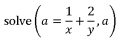Yes I know how to solve it manually a=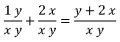But how can I get the TI-36 calculator to do it? I've tried using its Numeric equation solver but got an Invalid Domain Error so I'm not doing it correctly?

Edited to remove ambiguity

Last edited:

#### WBahn

Joined Mar 31, 2012
27,862
I'm not familiar with the notation you are using.

When you say

solve( a = f(x,y), a)

What, exactly does that mean? What is it that you are solving for?

•MrSalts

#### SamR

Joined Mar 19, 2019
4,484

#### DickCappels

Joined Aug 21, 2008
9,508
With Mathematica the expression says to solve for a.

#### SamR

Joined Mar 19, 2019
4,484
Here is the initial expression in MS Mathematics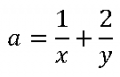Which it then translates toWhich it then solves as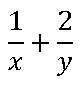Right back where we started! What I am trying to get it to resolve to is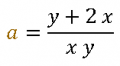Which MS Mathematics then solves as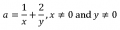I guess what I am looking for is the complex form of the simplified equation? Instead, it wants to simplify the simplified equation which it cannot do since it is already in the simplified form. The TI-36 has some solving abilities but it gives me an Invalid Domain Error. So, I was hoping someone had an answer as to how to get the complex form on the TI-36. Sometimes learning how to use the machine to save time takes longer than just doing it by hand!

#### WBahn

Joined Mar 31, 2012
27,862
I'm still unclear as to what it is that you are looking for.

How does a = (y+2x)/(xy) constitute any more of a solution to anything than a = 1/x + 2/y does?

Given the variables and the general form of the equation, this looks like the equations for a curve in the xy-plane given some parameter 'a'.

For instance, if we had a = x^2 + y^2, this would describe a circle having a radius of sqrt(a).

Looking at what you are given:

a = 1/x + 2/y

We see that neither x nor y can be zero.

We also see that if x = 1/a that y must be infinite and, similarly, if y = 2/a that x must be infinite.

So we have two asymptotes, a vertical one at x = 1/a and a horizontal one at y = 2/a.

We can then solve for y(x) and get

y = 2x/(ax-1)

If we plot this, we get the following for a = 5.

The vertical line at x = 1/a = 0.2 is an artifact of how the plot was generated. That should be a dashed line indicating the vertical asymptote. Furthermore, there should be a circle at the origin since the original function is not defined there.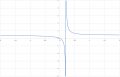#### SamR

Joined Mar 19, 2019
4,484
Not really. I'm not interested in plotting it and yes, MS Mathematics can do that. What I was looking for is, using the TI-36, MS Mathematics, or TI-nspire, how do I (if possible) use them to translate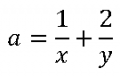into a single expression ofI'm sorta going backwards from simplified expression to complex expression which is the antithesis of what the calculators normally do and not covered in their documentation that I can find.

#### crutschow

Joined Mar 14, 2008
31,111
I'm sorta going backwards from simplified expression to complex expression
I doubt you will find any program to do that since, as you say, it's going backwards.

So why do you want to go backwards?
It's sort of like uncooking an egg.